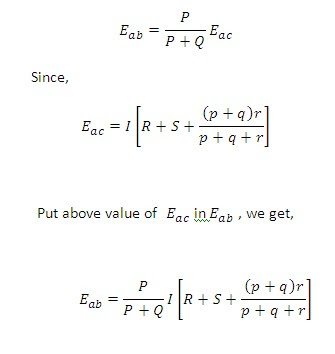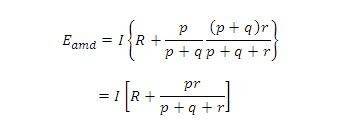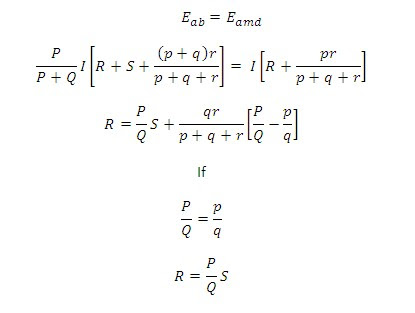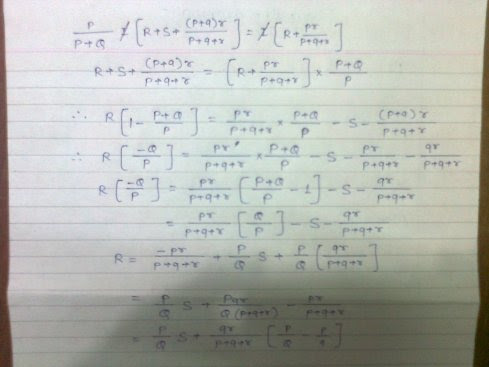# Kelvin double bridge circuit for measurement of low resistance

In this post we will see the Kelvin double bridge. It is used for the measurement of low resistances. The Kelvin double bridge is the modification of the Wheatstone bridge and provides greatly increased accuracy in measurement of low value resistance.

An understanding of the Kelvin bridge arrangement may be obtained by the study of the difficulties that arise in a Wheatstone bridge on account of the resistance of the leads and the contact resistances while measuring low valued resistance.

The Kelvin double bridge incorporates the idea of a second set of ratio arms-hence the name double bridge-and the use of four terminal resistors for the low resistance arms.

Figure shows the schematic diagram of the Kelvin bridge. The first of ratio arms is P and Q. the second set of ratio arms, p and q is used to connect the galvanometer to a point d at the appropriate potential between points m and n to eliminate effect of connecting lead of resistance r between the unknown resistance, R, and the standard resistance, S.

The ratio p/q is made equal to P/Q. under balance conditions there is no current through the galvanometer, which means that the voltage drop between a and b, Eab is equal to the voltage drop Eamd.

Now the voltage drop between a and b is given by,for bridge to be balanceAbove equation is the usual working equation for the Kelvin Double Bridge. It indicates that the resistance of connecting lead, r, has no effect on the measurement, provided that the two sets of ratio arms have equal ratio. The former equation is useful, however, as it shows the error that is introduced in case the ratios are not exactly equal. It indicates that it is desirable to keep r as small as possible in order to minimize the errors in case there is a difference between ratios P/Q and p/q.

solution:You may also like:

1.I do not understand the equation R=PQ/S, please explain details.

2.Some one knows the derivation of Eamd? How Eamd is drived?

3.Kelvin double bridge solution of derivation step by step send me?

4.R= variable resistance
PQ=standard resistance
S=unknown resistance